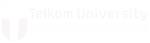# Statistics and Probability

START:
January 1, 2017
DURATION:
3 credits
ID:
MUG2D3
CREDIT:
3

This course provides students with the competence to understand the concept of probability and statistics quantities: the concept of probability such as sampling, probability, trial, set theory, conditional probability, and Bayes’ Theorem, independent events, random variables, pdf and cdf, expectation and variances. Distribution specials: uniform, Gaussian, binomial, Poisson, erlang. Introduction of random processes and modeling of random processes (state diagram), an introduction to statistics, and estimation of distribution.INTERNATIONAL OFFICE
TELKOM UNIVERSITY

Jl. Telekomunikasi Terusan Buah Batu No.1
Learning Center (Bangkit) Building R.118
Bandung, Jawa Barat 40257 Indonesia
F : +62-22-756-4108 ext 2400
M : +62-813-2112-3400
E : info@io.telkomuniversity.ac.id